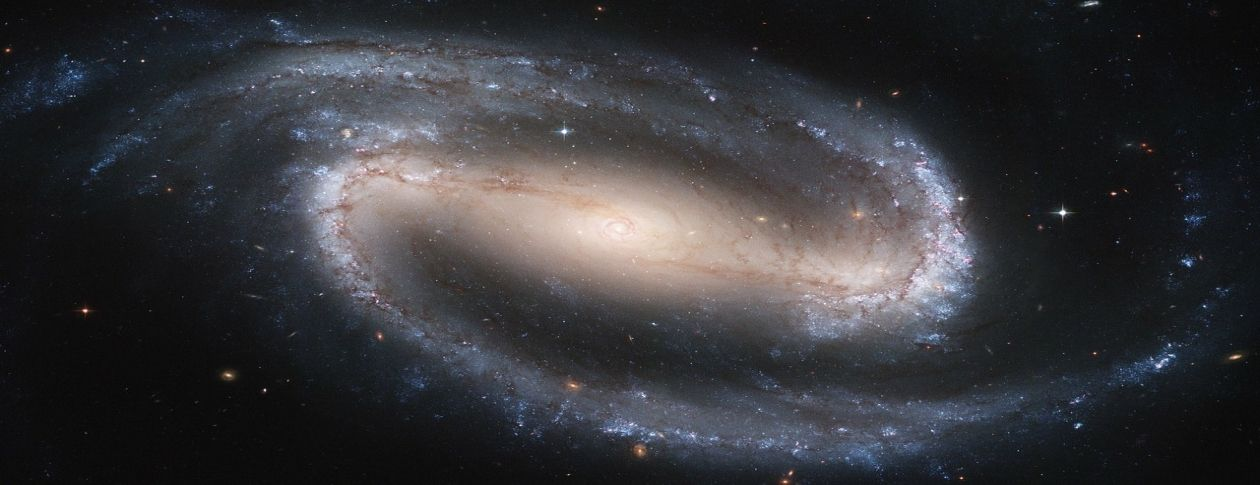## БЛОГWe consider a solution of the Einstein equations for a system comprised of merging black holes and show that the solution contains a term which represents a repulsive force. This repulsive term is proportional to 1/r, where r is the distance from the system, and it is additional to the common Newtonian term which is proportional to 1/r2. This repulsive force acts as an effective dark energy if the total mass of the Universe is decreased.

As seen below, a shape of the gravitational potential is often illustrated by a funnel made of rubber film where a heavy ball (blue) is located in the center. In this model, a fast decrease of the gravitational mass corresponds with a sharp ascent of the ball. The film attached to the ball forms a cone-type hill in the center of the funnel. Light balls (green) on the central cone run away from the center. The central cone expands fast but keeps it exterior slope; this corresponds to long-term repulsive force.

A mechanism of the repulsive force may be applied to a model of the expanding Universe. This may imply that the Big Bang and accelerated expansion of the Universe is not related to current processes in the Universe but to a relic repulsive gravitational force or to a configuration of space-time that originates in the previous cycle of the Universe when at the last stage of a collapse the intensive generation of gravitational waves resulted in sharp decrease of the gravitational mass of the Universe. This process generated a powerful repulsive force that transformed the Big Crunch into the Big Bang. Because the repulsive acceleration decreases with time, the current Universe expands with lower acceleration. Decreasing acceleration of the Universe can be verified by observations. Gravitational waves caused by the compression of the Universe may not disappear at the stage of expansion and form the relic gravitational radiation.

Read more